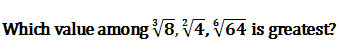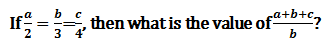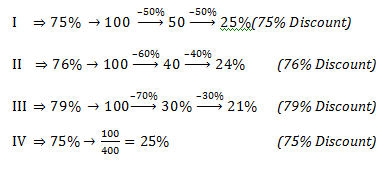# SSC Quantitative Aptitude Questions (Day-78)

Dear Aspirants, you can find the Quantitative Aptitude questions with detailed explanations for the SSC exams. Nowadays the competitive level of the exam has been increasing consistently. Due to the great demand for the government job, the level of the toughness reached greater. Candidates have to enhance the preparation process in order to drive in the right path. It doesn’t need to clear the prescribed cutoff. You must have to score good marks more than the cut off marks to get into the final provisional list. Here we have updating the Quantitative Aptitude questions with detailed explanations on a daily basis. You can practice with us and measure your level of preparation. According to that you can sculpt yourself in a proper way. SSC aspirants kindly make use of it and grab your success in your career.

Start Quiz

1) How many factors of 108 is/are prime number?

(a) 2

(b) 3

(c) 5

(d) 6

Direction (2-3): Which of the following expression(s) is/are true?

2) I) √5 + √5 > √2 + √8

II) √3 + √7 > √2 + √8

III) √5 + √5 > √3 + √7

(a) Only I

(b) Only I and III

(c) Only II

(d) All expressions are true

3) I)
337 is a prime number.

II) The number 12 has 6 positive factors.

III) 32724 is completely divisible by 9.

(a) Only I

(b) Only I and II

(c) Only II and III

(d) All expressions are true

4)(a)∛8

(b) √(2&4)

(c) √(6&64)

(d) All are equal

5) What is the value of (1004)2 – (998)2?

(a) 11012

(b) 12012

(c) 120012

(d) 1212

6) A and B together can complete a work in 15 days. They started together but after 5 days A left the work. If the remaining work is completed by B in 15more days, then A alone can complete the entire work in how many days?

(a) 25

(b) 50

(c) 35

(d) 45

7) P is 20% more efficient than Q. If Q alone can finish a work in 10 days, then in how many days P alone will finish the work?

(a) 8 1/3

(b) 8.5

(c) 9

(d) 12

8) The ratio of marked price and cost price of an article is 6 : 5. If 15% discount is given, then how much is the profit (in percent)?

(a) 4

(b) 0.2

(c) 2

(d) 1

9) Out of the following, which is the best discount for a customer?

I) 50% + 50% off

II) 60% + 40% off

III) 70% + 30% off

(a) Only I and IV

(b) Only II

(c) Only I, II and III

(d) Only III

10)(a) 3

(b) 2

(c) 4/3

(d) 7/3

108 ⇒ 2², 3³

Two factors are prime.

√7 + √3 & √5 + √5  and √2 + √8

Squaring all terms

10 + 2√21, 10+2√25, 10 + 2√16

Hence

√5 + √5 > √7 + √3 > √2 + √8

I — 337 is a prime

II — 12 = 2².3

(3 × 2) = 6 factors

III — 3 + 2 + 7 + 2 + 4 = 18

∵ sum of digits is divisible by 9

Hence no. is divisible 9.

∛8 √(2&4)√(6&64)

8 = 2³, 4 = 2², 64 = 2⁶

Hence all are equal.

(1004)² – (998)²

= (1004 –998) (1004 + 998)

= 12012

In 5 days A & B will work ⅓ of the given work

& to finish ⅔rd of the given work B takes = 15 days

Hence, B will finish in = 45/2 days

1/A = 1/15 – 2/45

= (3–2) / 45

= 1/45

A = 45 days

Suppose if Q work 5 units per day

Then P will do = 6 units per day (20% efficient)

Total work = 10 × 5 ⇒ 50 units

P will finish in 50/6 days

MP/CP = 6/5

Let MP = 600 and & C.P. = 500

For 15% discount ⇒ 600× 15/100 = 90 Rs.

S.P. = 600 – 90 = 510

Profit % = (510 –500) / 500 × 100%

= 2%